2022 NFL Offensive Rookie of the Year Odds
+500
5 to 1Kenny Pickett
8.2% implied probability

+750
7.5 to 1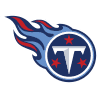Treylon Burks
5.8% implied probability

+800
8 to 1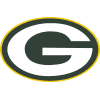Christian Watson
5.4% implied probability

+800
8 to 1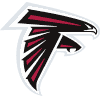Drake London
5.4% implied probability

+900
9 to 1Breece Hall
4.9% implied probability

+1000
10 to 1Chris Olave
4.4% implied probability

+1000
10 to 1Skyy Moore
4.4% implied probability

+1000
10 to 1Garrett Wilson
4.4% implied probability

+1200
12 to 1Kenneth Walker
3.8% implied probability

+1200
12 to 1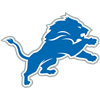Jameson Williams
3.8% implied probability

+1400
14 to 1Desmond Ridder
3.3% implied probability

+1400
14 to 1James Cook
3.3% implied probability

+1800
18 to 1Jahan Dotson
2.6% implied probability

+1800
18 to 1Matt Corral
2.6% implied probability

+2000
20 to 1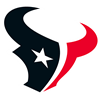Dameon Pierce
2.3% implied probability

+2000
20 to 1Malik Willis
2.3% implied probability

+2500
25 to 1Sam Howell
1.9% implied probability

+2500
25 to 1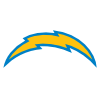Isaiah Spiller
1.9% implied probability

+3000
30 to 1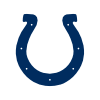Alec Pierce
1.6% implied probability

+3000
30 to 1Jalen Tolbert
1.6% implied probability

+3000
30 to 1Rachaad White
1.6% implied probability

+3500
35 to 1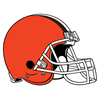David Bell
1.4% implied probability

+4000
40 to 1Carson Strong
1.2% implied probability

+4000
40 to 1George Pickens
1.2% implied probability

+4000
40 to 1Kyren Williams
1.2% implied probability

+4000
40 to 1Trey McBride
1.2% implied probability

+4000
40 to 1Justyn Ross
1.2% implied probability

+5000
50 to 1Tyler Allgeier
1.0% implied probability

+5000
50 to 1John Metchie
1.0% implied probability

+5000
50 to 1Tyquan Thornton
1.0% implied probability

+6000
60 to 1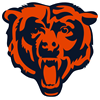Velus Jones
0.8% implied probability

No changes have been recorded yet.
+6000
60 to 1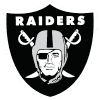Zamir White
0.8% implied probability

+7000
70 to 1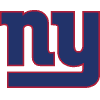Wan'Dale Robinson
0.7% implied probability

+10000
100 to 1Isaiah Likely
0.5% implied probability

+10000
100 to 1Cade Otton
0.5% implied probability

+10000
100 to 1Zonovan Knight
0.5% implied probability

+10000
100 to 1Calvin Austin
0.5% implied probability

+10000
100 to 1Romeo Doubs
0.5% implied probability

+10000
100 to 1Hassan Haskins
0.5% implied probability

+10000
100 to 1Dontario Drummond
0.5% implied probability

+10000
100 to 1Pierre Strong
0.5% implied probability

+10000
100 to 1Jeremy Ruckert
0.5% implied probability

+10000
100 to 1Kyle Phillips
0.5% implied probability

+10000
100 to 1Tyler Goodson
0.5% implied probability

+10000
100 to 1Jerome Ford
0.5% implied probability

+10000
100 to 1Jerrion Ealy
0.5% implied probability

+10000
100 to 1Ty Chandler
0.5% implied probability

+10000
100 to 1Bailey Zappe
0.5% implied probability

+10000
100 to 1Kaleb Eleby
0.5% implied probability

+10000
100 to 1Jack Coan
0.5% implied probability

+10000
100 to 1Jalen Wydermyer
0.5% implied probability

+10000
100 to 1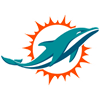ZaQuandre White
0.5% implied probability

+10000
100 to 1Charles Cross
0.5% implied probability

+10000
100 to 1Khalil Shakir
0.5% implied probability

+10000
100 to 1Tyler Linderbaum
0.5% implied probability

+10000
100 to 1Evan Neal
0.5% implied probability

+10000
100 to 1Ikem Ekwonu
0.5% implied probability

+10000
100 to 1Brian Robinson
0.5% implied probability